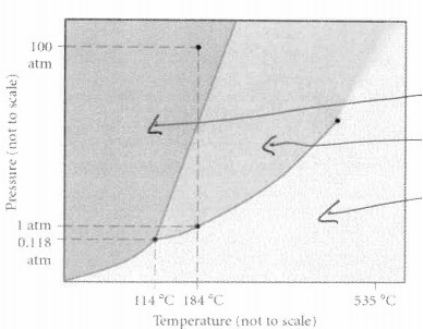Study Guides (390,000)
CA (150,000)
UTSC (10,000)
Chemistry (200)
CHMA11H3 (100)
all (30)
Midterm

# CHMA11H3 Study Guide - Midterm Guide: Trigonal Planar Molecular Geometry, Boiling Point, Molecular Geometry

Department
Chemistry
Course Code
CHMA11H3
Professor
all
Study Guide
Midterm

This preview shows pages 1-2. to view the full 6 pages of the document.
Multiple choice. Choose the one alternative that best completes the statement or
1) What is the molecular geometry of ClF4-?
a) Seesaw B) tetrahedral
c) square planer D) Square pyramidal
2) Using the VSEPR model, the geometry of the electron groups around the central atom in
SF4 is
a) Octahedral
b) Trigonal planar
c) Linear
d) Linear
e) Trigonal bipyramidal
3) Determine the vapor pressure (in mmHg) of a substance at 29oC , whose normal boiling
point is 76oC and has a Hvap of 38.7 kJ/mol. You may use the Claussius-Clapeyron
equation:
Ln(P2)-ln(P1) = -Hvap / R [1/T2 – 1/] and note that 1 atm = 760 mmHg.
4) Which is expected to have the largest dispersion forces?
A) F2B) C12H26 C) BeCl2d) C3H8
5) Consider the phase diagram below. If the dshed line at 1atm of pressure is followed from
100 to 500 oC, what phase changes will occur(in order of increasing temperature)?

Only pages 1-2 are available for preview. Some parts have been intentionally blurred.A) Condensation, followed by vaporization
B) Sublimation, followed by deposition
C) Melting, followed by vaporization
D) Vaporization followed by deposition
E) No phase change will occur under the conditions specified
6) How much energy must be removed from a 125 g sample of benzene (molar mass=
78.11 g/mol) at 425.0K to liquify the sample the sample and lower the temperature to
335.0k? the following physical data may be useful.
Hvap = 33.9kJ/mol
Hfus = 9.8kJ/mol
Cliq = 1.73 J/goC
Cgas = 1.06 J/goC
Csol= 1.51 J/goC
Tmeling = 279.0 K
Tboiling = 353.0 K
a) 95.4kJ b) 67.7 kJ C) 54.3 kJ D) 74.4 kJ E) 38.9 kJ
7) Indentify the compound that does not have dipole-dipole forces as its strongest force.
a) CH2Cl2
b) CO2
c) CH3Br
d) CH3OCH3